## 9 September 2014

### Introducing Differentiation

A while ago I was asked by @_JPMason to write a post about how I introduce differentiation to my Year 12s. I've been procrastinating for weeks but here I am, large glass of wine in hand, attempting to tackle this challenge. I've only introduced calculus a few times and I don't think I've ever done it particularly well. So this is a good opportunity for me to do some research and some thinking which will hopefully improve my own teaching practice.

This post is primarily aimed at teachers who are teaching calculus for the first time - I hope you find some useful ideas here.

An Instantaneous Rate of Change
As well as knowing how to differentiate, students need an intuitive understanding of the gradient of a curve at a point.

Students are familiar with the concept of gradient as a rate of change, but only in the context of the steepness of straight lines. Their next step is to understand that they can also determine the gradient of a curve. But unlike a straight line, the steepness of a curve changes constantly. Hence we have a gradient function - put in a value of x and we find the derivative at a specific point - an 'instantaneous rate of change'.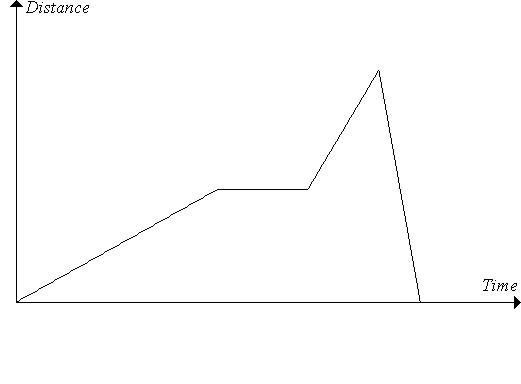You could start by showing your students a simple distance-time graph. Discuss how the gradient changes in each section of the graph - your students will easily identify positive, negative and zero gradients. Ask them how to assign a number to those gradients and they will probably recall that gradient is calculated as 'rise over run' ie distance divided by time. In a distance-time graph, the value of the gradient represents speed. Now discuss the idea that straight lines represent constant speed. Ask your students if they can run or cycle at a constant speed. Perhaps try it in the classroom - have your students walk across the room simultaneously, attempting to maintain constant speed. Impossible? If your school is lucky enough to own a motion sensor then this is a good opportunity to use it - graph matching activities are great fun. The point is that speed varies and therefore it should be represented by a curve. It's easy to determine the gradient of a straight line but how can we determine the gradient of a curve? That's the big question.'Making sense of differentiation' by Project Maths describes the idea of an instantaneous rate of change in a way your students will understand: "The speed of an object at an instant in time is surprisingly difficult to define precisely. Consider the statement: 'At the instant he crossed the finish line in 2009 Usain Bolt was travelling at 28 mph'. How can such a claim be substantiated? A photograph taken at that instant would be no help at all as it would show Usain Bolt motionless. There is some paradox in trying to study Usain’s motion at a particular instant in time since, to focus on a single instant, you effectively stop the motion! Problems of motion were of central concern to Zeno and other philosophers as early as the 5th century B.C. The modern approach, made famous by Newton's calculus, is to stop looking for a simple notion of speed at an instant, and instead to look at speed over small time intervals containing the instant". Khan Academy has a really good Introduction to Differential Calculus video about Usain Bolt and instantaneous rates of change.

Once you've given students a taste of what calculus involves, try a simple activity like this to get them thinking about the changing gradients of curves: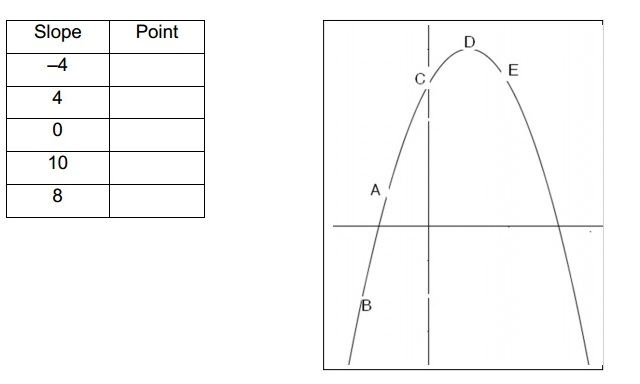Source: Project Maths
They should be able to complete the table without any instruction, based on what they know about gradients from Key Stage 3/4. I've produced a worksheet that covers these introductory ideas. This could be be set as a homework in advance of the first calculus lesson.

First Principles
Once students start differentiating using a set of rules, this topic is fairly straightforward. They apply a simple procedure and get the answers right - hey presto, they're doing calculus. But it's essential that we show them where the rules come from, so let's look at that.

Like most A level teachers, my first calculus lesson focuses on differentiation from first principles. In the great calculus controversy of the 17th Century, Newton and Leibniz independently invented calculus to allow the notion of rate of change to be made mathematically precise. To quote Jonny Griffiths, "I like the idea that as mathematics teachers we are trying to rerun the history of the subject inside our students' heads, only quicker! Maybe discovering the rule for differentiating xn is such an important event in this history that this activity wins its place in an AS course where time is tight".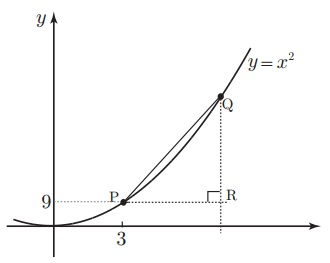In my lesson on differentiating from first principles, I plot y = x2 on the board and approximate the gradient at x = 3 using a series of increasingly short chords. Once we've established that the gradient of the tangent (and hence the curve) at this point is 6, I do the same for a couple more points. I then go through the algebra for the general point (x, x2). We establish that the gradient function is 2x and talk about how we can use that function to find the gradient at any point.

Then I get the students to have a go with y = x3 and y = x4. They look for patterns and figure out the rule for y = xn. Sounds straightforward but the limits and deltas may confuse your students, so don't rush them.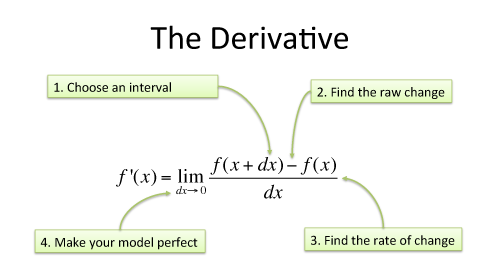betterexplained.com

This first principles method is clearly explained in this article from Math Centre. If you haven't taught a first principles lesson before and are a bit unsure then watch this video to see how it's explained. Most teachers I've spoken to introduce differentiation in a similar way. Some do a lot less explaining than me, instead getting the pupils to do most of the work themselves by hand or using ICT. If you're looking for a resource to guide you in this first principles lesson then this worksheet from SRWhitehouse on TES might be helpful. If you'd rather students use ICT (Desmos or Autograph), then this RISP gives some guidance.

Resources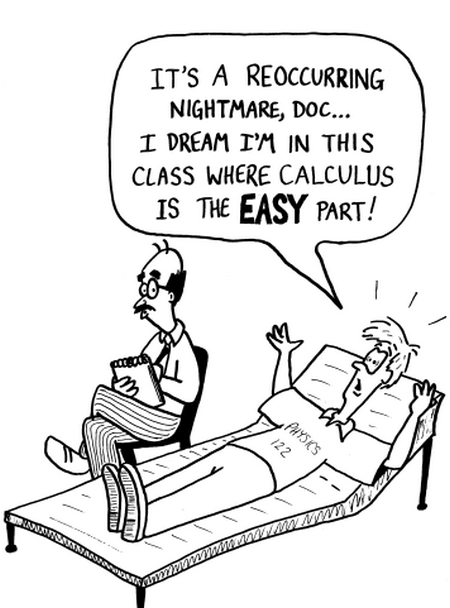I really enjoy teaching differentiation once we've got past that tricky first lesson. Optimisation is particularly satisfying.

I've listed a few teaching resources here.

For activities that help students practise the basic rules, there are simple polynomials dominoes and factorised quadratic dominoes from Teachit Maths. WJEC gives us a differentiation pelmanism for the interactive whiteboard and the Standards Unit has a Matching Functions and Derivatives activity.

SRWhitehouse on TES gives us selection of excellent differentiaton exercises.

Once you get onto stationary points, here's a few resources to try: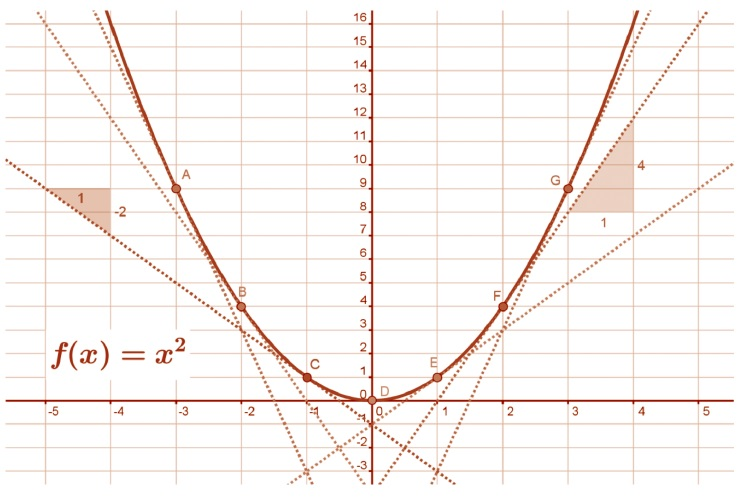Project Maths is a fantastic website with lots of good resources, many of which are featured in my resource libraries. It’s based on the Irish school system so is a bit hard to navigate if you’re not familiar with their curriculum. The best bits are the activities at the back of their T&L plans - for example Introduction to Calculus is packed full of excellent resources for introducing differentiation and explaining concepts relating to rates of change. It even features a Usain Bolt activity which ties in nicely with the ideas above.

Final thoughts
Differentiation really exposes gaps in students' indices and algebra skills. If you're looking for resources to help you teach the other topics covered in Year 12, see my Core AS Resources Library.

I highly recommend that all maths teachers read the articles about calculus on betterexplained.com, starting with Calculus: Building Intuition for the Derivative.Jo or Gottfried?
Calculus is a rare branch of mathematics in which you actually want a student to ask the question 'But Miss, when will we ever need to know this?'. There are endless applications in a wide number of fields including engineering, architecture, medicine, physics, finance and economics.

As usual, I'm really keen to hear your teaching ideas - please comment below or tweet me.

Apologies for any mistakes in my terminology in this post. I'm no Leibniz. If I've made any glaring errors then do let me know.

If all else fails then ask your students to buy the Cartoon Guide to Calculus. Who needs teachers?